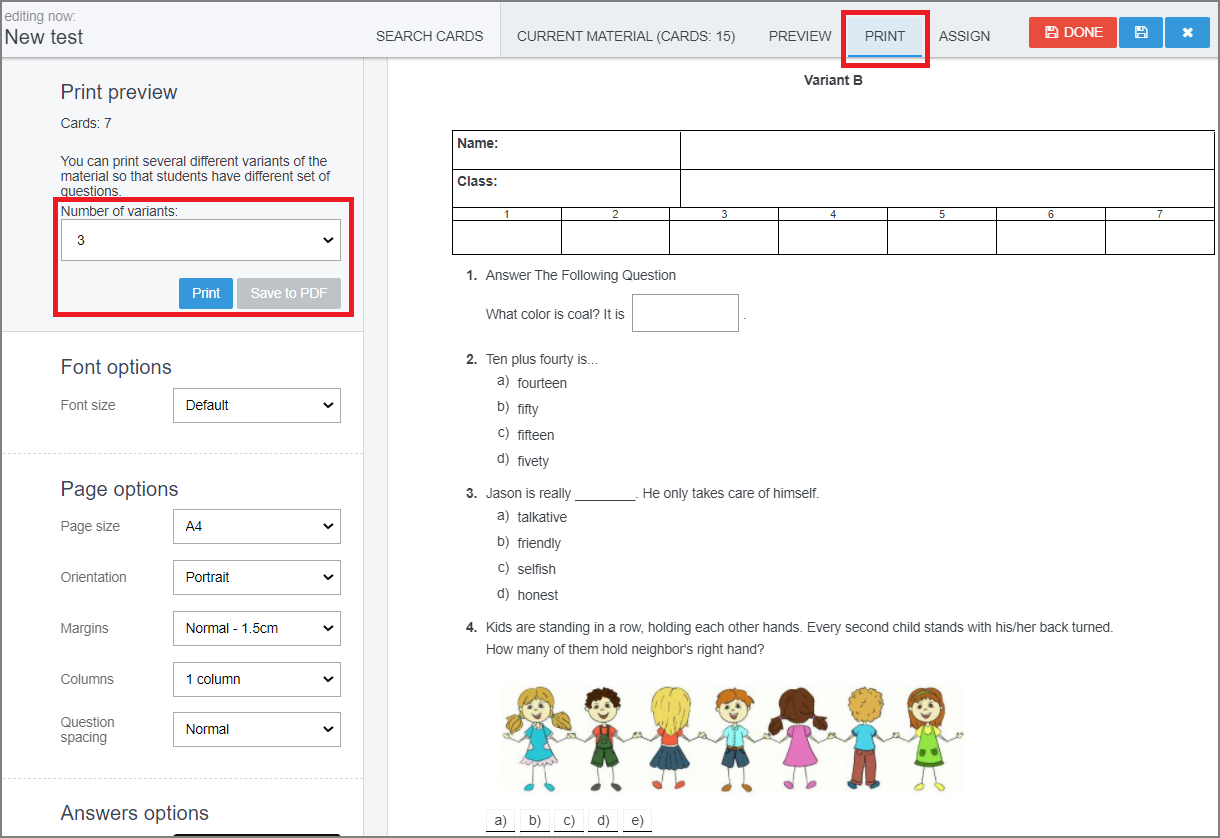EN# Versions of printed tests

When the teacher prepares the test for students to elaborate on paper, various printed versions can be created (e.g. group A, group B, group C). The versions will vary in the order of questions and e.g. in order of the order of answers in ABCD questions.

In the 'Print' section select the number of variants of the test. The system generates the desirable number of versions that you can print out or save to PDF.You can evaluate the printed tests generated in EduPage: How to evaluate printed tests?

If you create the test with the questions from question groups then the system picks one question to each student from the groups randomly. Each student receives different set of questions. More information about generating versions of printed tests from question group and evaluating such tests: Versions of printed tests with question groups